# Catch up on the latest AI articles# Let's Survey The Optimization Algorithm And Understand The Tendency!

3 main points
✔️ One of the benchmarks for large-scale optimization algorithms was proposed.
✔️ Optimization algorithms depend on the problem.
✔️ Default use may be useful.

Descending through a Crowded Valley -- Benchmarking Deep Learning Optimizers
w
(Submitted on 9 Mar 2020)

Subjects: Machine Learning (cs.LG); Machine Learning (stat.ML)
Paper Official Code
COMM Code

## Introduction

We all know that optimization algorithms play an important role in the training time and accuracy of a learning model. Many people are currently trying their best to increase the accuracy of the learning model as much as possible by choosing the optimization algorithm and tuning its hyperparameters. However, there is no clear result of the analysis such as the selection of the optimization algorithm or tuning of the hyperparameters, and it is mostly done by feeling or practical experience. Also, the research on the optimization algorithm is increasing year by year due to its large impact on accuracy. Figure 1 shows the papers and annual trends for optimization algorithms, and it is safe to assume that the number of papers and annual trends for optimization algorithms is basically increasing, as the number appears to be decreasing from 2019 to 2020 simply because the collection period is still in 2020.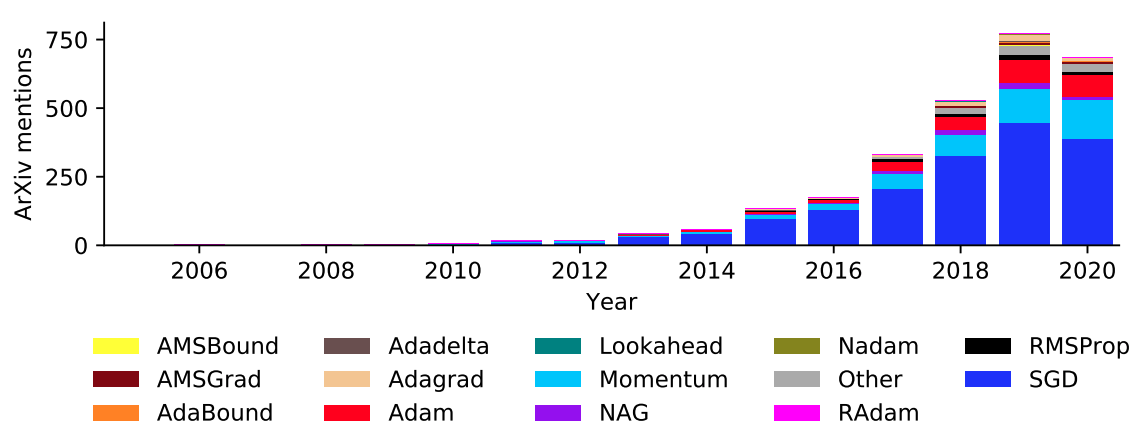Figure 1. Papers and annual trends for the optimization algorithm.

It is also common for most of the proposed studies of optimization algorithms to show that the researcher's own optimization algorithm is dominant. Do you know how many optimization algorithms are currently available? A survey table 1 of the authors' optimization algorithms is presented. This still does not cover everything. Table 2 also summarizes Table 2, which provides an overview of the parameter schedule of the optimization algorithm.

Table 1: Types of optimization algorithms.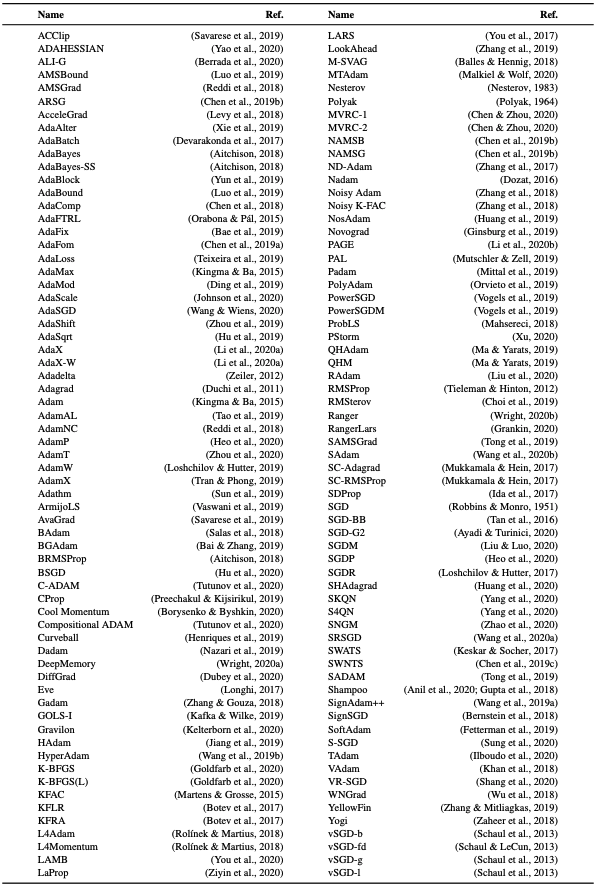Table 2. Summary of Optimization Algorithm Schedule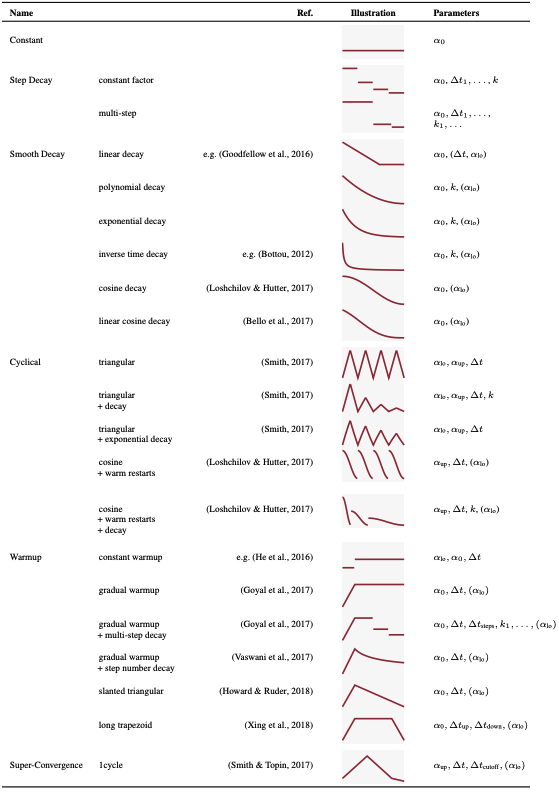I think it may surprise some people, but at least this is all that has been suggested. The tricky part is that these comparative analyses are few and far between. That's why most people tend to use the old favorites like SGD. Why do we never do comparative analysis? It is because the combination of items to be considered in these comparative analyses is enormous. In addition to this, there are large time and labor costs due to repeated adjustments and experiments, as well as computational resource issues.

So, the paper presented here is a fundamental study that compares the impact of optimization algorithm choices and hyperparameters to get a sense of trends in optimization algorithms. (One caveat: the authors don't have unlimited resources either, so it's best to think of it as only a practical range of study. Also, this is a start and not yet a study of the entire model, so it's still sweet, but I think it's just good for catching trends and looking at the entire optimization algorithm from an overhead perspective).

Contribution

• Extensive summary catch-up of the optimization algorithm is possible (Tables 1 and 2)
• Proposed one of the benchmarks for large scale optimization algorithms
• With thousands of analyses and empirical experiments, the optimization algorithm depends on the problem
• There is often no significant difference between the default parameters of the optimization algorithm and the tuning parameters (see the article "Parameter tuning is black magic of experience differences! " in AI-SCHOLAR). But there are suggestions for handling default values for the same parameters.)
• Learning rate schedules are relatively more effective than "unadjusted" schedules, but their effectiveness varies widely by problem
• No generalized optimization algorithm was found from the experiments, but on the contrary, a problem-specific optimization algorithm was found to be

## Experiment

The experiment is simple. See Figure 2.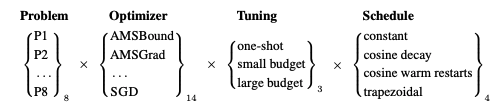Figure 2. Considerations and combinations

• Evaluating optimization algorithms for 8 (P1~P8) problems
• 14 most popular optimization algorithms were chosen.
• In contrast, there are three tunings.
• Considering four learning rate schedules

We will use a combination of these measures. We use multiple evaluation metrics rather than one specific one for research, but since we are assuming AI engineers in the real world, we are only referring to the evaluation in terms of test accuracy (or test loss) in this case.

### Problem

Each task is summarized in Table 3.

Table 3. 8 Problems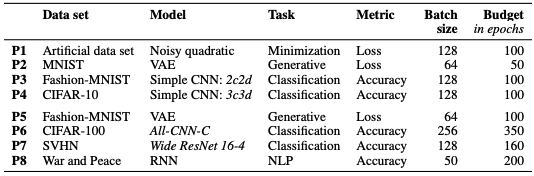P1~P4 questions are small data sets, P5~P8 are large data sets. Models, tasks, etc. are as shown in the table.

### Optimizer

Among the many optimization algorithms, we have selected 14 popular optimization algorithms in the sense that they are the most used. See Table 4 for details. In particular, Tuning Distribution is the search range for adjusting parameters during the next tuning session.

Table 4. Each of the selected optimization algorithms.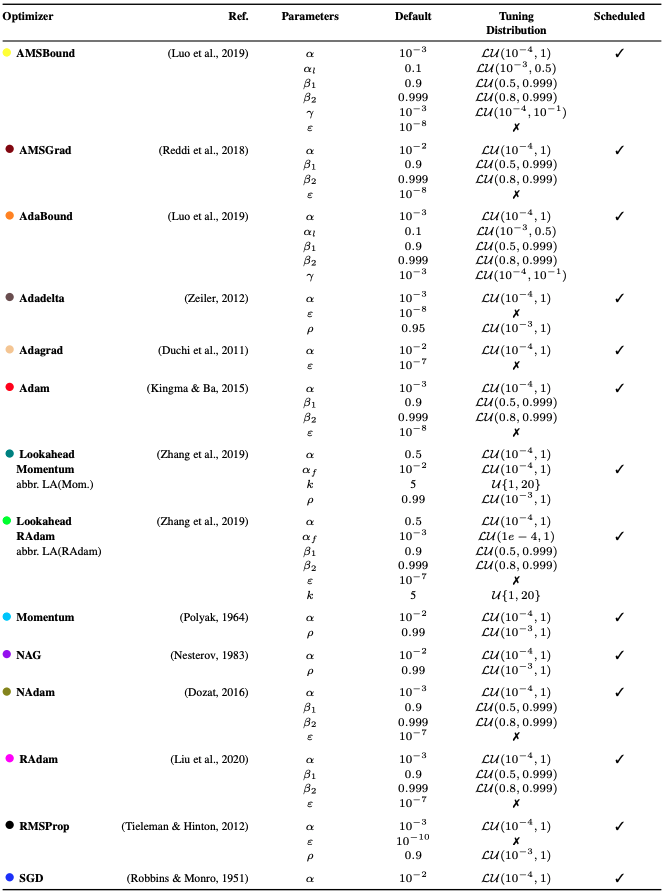### Tuning

There are three ways to tune it.

1. The default values by the proposer and researchers are used. Please refer to Table 4 in the previous section for default values.
2. Small Budget Tuning (small budget)
3. Large resource tuning (large budget)

Small and large budgets

Tuning is performed 25 times (small budget) and 50 times (large budget) by using only a single seed. After that, since the influence of accuracy by the seed may occur by chance, we randomly set 10 seed values with the best-tuned setting, and evaluate them by the average value and standard deviation. A random search is used for tuning.

### Scheduled

In terms of the learning rate schedule, we set four different schedules: constant, cosine decay, cosine with warm restarts, and trapezoidal. You can see the details in Table 2.

## Result

The number of experiments on this issue is so great that there are many results. I will focus on some of the most unique ones. If you are interested in the other results, please take a look at the original book. I think you'll be overwhelmed by the sheer volume of results.

Figure 3 below shows the results.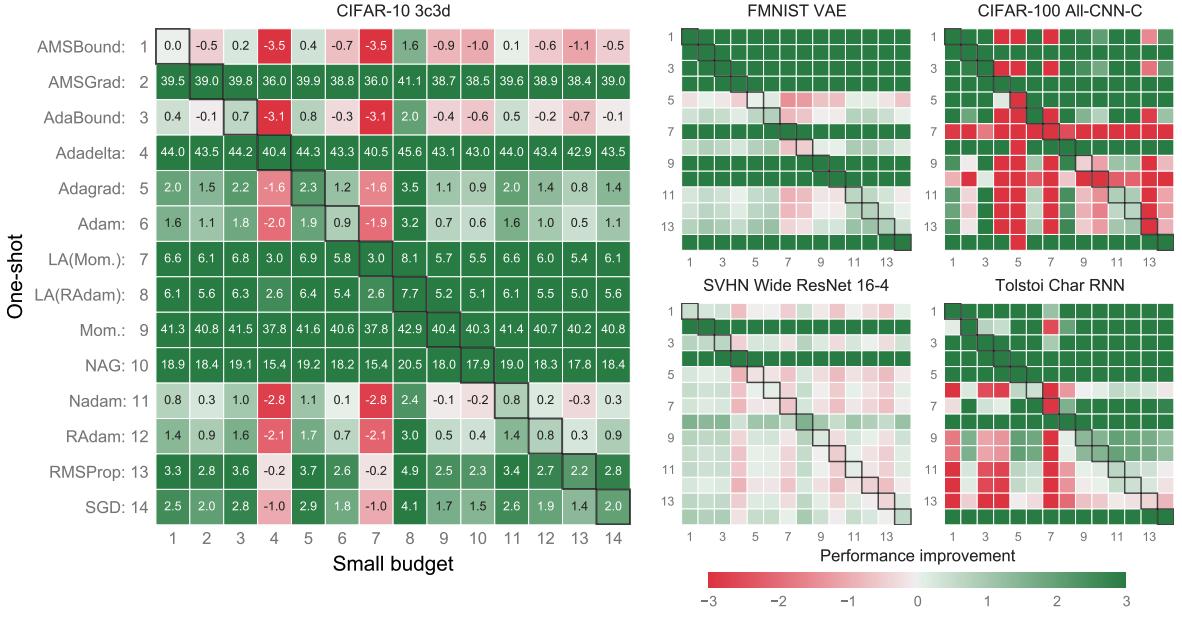Figure 3. Results (differences due to tuning)

Those that have been improved are shown in green and those that have not been improved are shown in red. For example, if we look at the problem: CIFAR-10, model: 3c3d (far left), and the bottom left cell, we can see that AMSBound(1)(Small budget) is more than 2.5% more accurate than SGD(14)(One-shot: using default values). (Basically, look at the optimization algorithm column vertically.)

In a more straightforward way, Adadelta(4)(Small budget) is inferior to the default use of most optimization algorithms if you look at it vertically (red). However, it seems to be more accurate than One-Shot's 7, 8, 9 , and 10. So on a horizontal basis, we can see that AMSBound(1)(One-shot: using default values) is more accurate than most tuned optimization algorithms, since red means better accuracy.

The results for the problem criteria are shown in Figure 4.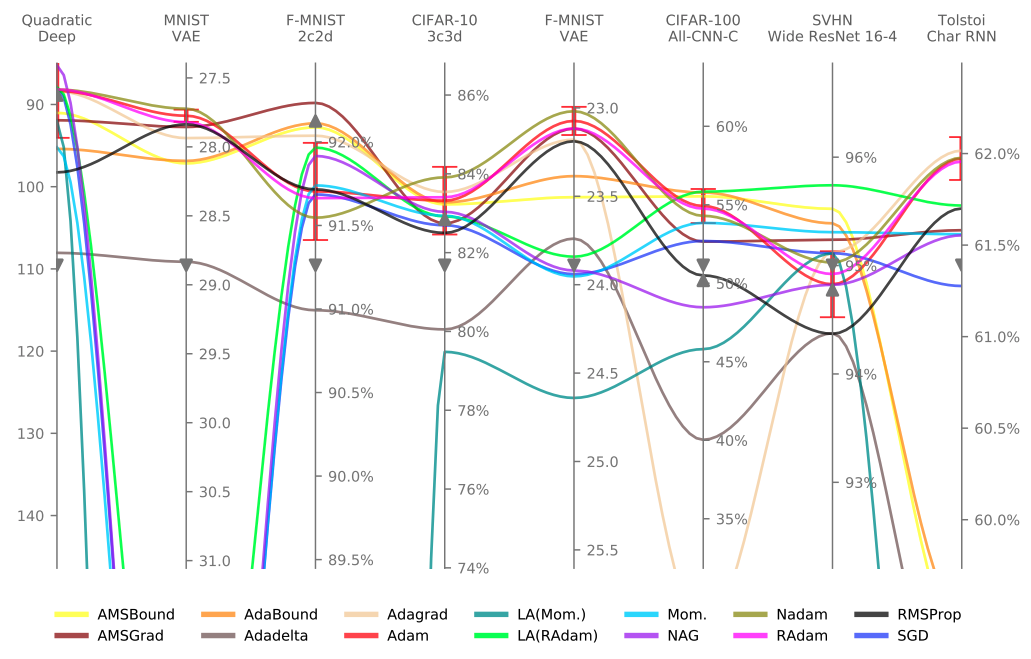Figure 4. Results (differences by problem)

It is highly dependent on the task as there is a large difference in performance. It shows that there is an optimization algorithm.

## Summary

This review has not yet been fully explored.

• Do models of different complexity and magnitude yield the same results?
• The trend of this optimization algorithm may change depending on the model.
• The results may be more generalized by addressing DEEPOBS, which includes a wide range of deep learning test questions along with a standardized procedure for evaluating optimization algorithms.
• This may change depending on the framework.
• It is possible that this consideration may not be generalizable to something that takes large resources like GAN or reinforcement learning.
• Differences in parameter search methods are also an item to consider.
• Reproducibility Stability

There is still a lot of work to be done in this way. However, there is a good chance that the results of this study will provide a useful first look at these practical society engineers. Also, this study may provide an opportunity to propose new algorithms as well as new ways to use them.

If you're going to make adjustments, including an optimization algorithm

1. Select the optimization algorithm to be considered once ←This is important because of the dependency between the task and the optimization algorithm (but there is no clear selection method).
2. Basically, a generic one, using default values
3. Changing the learning rate schedule←Beware of problems that can severely affect the accuracy

This is the only way to tell from this discussion. But it is recommended to use the default values or common ones that are used relatively. It is not so simple, but it is better for reproducibility to compare all the default values than to compete with the fine-tuning.

If you have any suggestions for improvement of the content of the article,